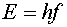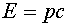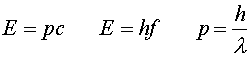Photons as light quanta

A photon is a quantum of light. Our picture of light up to this point has been that of a wave, and wave-like characteristics are indeed clearly demonstrated by interference and diffraction effects. However, light is absorbed and emitted one photon at a time. The energy of a photon is related to the frequency of the light wave by Planck's constant:Photons are massless particles. The idea of a massless particle may seem a bit strange. To understand it better, first consider the expressions for energy and momentum of particles with mass m.Massless particles can be thought of as the limit of massive particles with m approaching zero. Even though the mass may be infinitesimally small, the factor g may approach infinity as the velocity approaches c. Thus for massless particles, the above expressions are meaningless. However, the ratio of the two expressions is valid even for zero mass because the factors g and m cancel.We have used the fact that the velocity v equals c for massless particles. Bringing in the relation between wavelength and frequency, c = l f, you can get three useful expressions relating energy, momentum, frequency and wavelength:Thus light of shorter wavelengths interacts in the form of photons with higher energy than light of longer wavelengths. Later we will discuss the fact that the last of these three formulas is also true for particles with non-zero mass.

Quantum physics index      examples        Lecture index